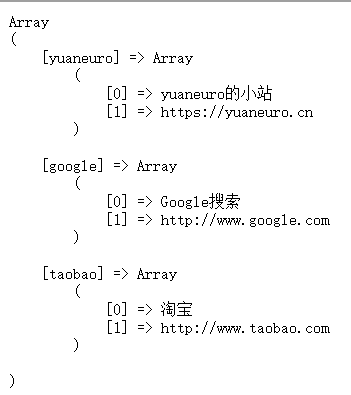## php定界符EOF

<?php
$name="yuaneuro";$a= <<<EOF
"abc"$name "123" EOF; // 结束需要独立一行且前后不能空格 echo$a;
?>

"abc"yuaneuro
"123"

• 结束标识必须顶格独自占一行(即必须从行首开始，前后不能衔接任何空白和字符)。
• 在 PHP 定界符 EOF 中的任何特殊字符都不需要转义；

## PHP 类型比较

• 松散比较：使用两个等号 == 比较，只比较值，不比较类型。
• 严格比较：用三个等号 === 比较，除了比较值，也比较类型。

#### 在ctf中有一种很常见的题目：

if (isset($_GET['a']) and isset($_GET['b'])) {
if ($_GET['a'] !=$_GET['b'])
if (md5($_GET['a']) === md5($_GET['b']))
die('Flag: '.$flag); else print 'Wrong.'; } 这里要求传入的a和b不相等，且md5值相等，就可以利用php弱类型绕过 payload：?a[]=1&b[]=2 ## PHP 常量 设置常量，使用 define() 函数，函数语法如下： bool define ( string$name , mixed $value [, bool$case_insensitive = false ] )

• name：必选参数，常量名称，即标志符。
• value：必选参数，常量的值
• case_insensitive ：可选参数，如果设置为 TRUE，该常量则大小写不敏感。默认是大小写敏感的。

<?php
define("yuaneuro", "欢迎访问 yuaneuro.cn");
function myTest() {
echo yuaneuro;
}
myTest();    // 输出 "欢迎访问 yuaneuro.cn"
?>

• 常量是全局的
$txt2="What a nice day!"; echo$txt1 . "yuaneuro " . $txt2; ?> 就会输出：Hello world!yuaneuro What a nice day! ## 三元运算符 • (expr1) ? (expr2) : (expr3) 对 expr1 求值为 TRUE 时的值为 expr2，在 expr1 求值为 FALSE 时的值为 expr3。 • 在php5.3+中表达式 expr1 ?: expr3 在 expr1 求值为 TRUE 时返回 expr1，否则返回 expr3。 • 在 PHP7+ 版本多了一个 NULL 合并运算符 ?? $username = $_GET['user'] ?? 'nobody'; 如果$_GET['user'] 不存在返回 'nobody'，否则返回 $_GET['user'] 的值 ## 组合比较符(PHP7+) 也称之为太空船操作符，符号为 <=> 语法 $c = $a <=>$b;

• 如果 $a >$b, 则 $c 的值为 1。 • 如果$a == $b, 则$c 的值为 0。
• 如果 $a <$b, 则 $c 的值为 -1。 ## 遍历数值数组 可以使用 for 循环 <?php$cars=array("Volvo","BMW","Toyota");
$arrlength=count($cars); //获取数组的长度

for($x=0;$x<$arrlength;$x++)
{
echo $cars[$x]; //ID 键
echo "<br>";
}
?>

## 关联数组

• 关联数组是使用您分配给数组的指定的键的数组

$age=array("Peter"=>"35","Ben"=>"37","Joe"=>"43"); 调用则可直接使用$age["Peter"]即可

## 遍历关联数组

<?php
$age=array("Peter"=>"35","Ben"=>"37","Joe"=>"43"); foreach($age as $x=>$x_value)
{
echo "Key=" . $x . ", Value=" .$x_value;
echo "<br>";
}
?>

## 多维数组

<?php
$sites = array ( "yuaneuro"=>array ( "yuaneuro的小站", "https://yuaneuro.cn" ), "google"=>array ( "Google搜索", "http://www.google.com" ), "taobao"=>array ( "淘宝", "http://www.taobao.com" ) ); print("<pre>"); // 格式化输出数组 print_r($sites);
print("</pre>");
?>## 其他

• 用户定义的函数和语言关键字对大小写不敏感。
• 声明常量使用关键字 define，例如
define(PI,3.14);

printf("PI = %.2fn",PI);

• \\ 是单引号字符串字面量中的一个特殊情况，它给出了一个单引号字符串字面量中没有插入\\n
• strpos() 函数查找字符串在另一字符串中第一次出现的位置，对大小写敏感。
• array_keys() 函数返回包含数组中所有键名的一个新数组，如果提供了第二个参数，则只返回键值为该值的键名。
Last modification：June 19th, 2020 at 07:01 pm